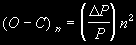from VSSC94

## Report On The Mira Variable R Aquilã Using BAAVSS Data

John Greaves

Observations for R Aquilµ from September 1908 to January 1997, amounting to 109 cycles of this Mira variable, were taken from the full 1899 to 1997 BAAVSS archive and separated into 9 sequential groups of exactly 12 cycles each (with 13 cycles in one instance where a maximum had been completely missed due to seasonality). Unfortunately there was a complete gap in the archive for the years 1934 to 1938 inclusive which would have meant having to start the investigation from 1939. In order to avoid this problem, and further so as not to waste the excellent data between 1908 and 1934, this interregnum was filled via the AFOEV public domain archive at the CDS, Strasbourg1. An average period was derived for each of these cycle groups with software utilising the Phase Dispersion Method2 as shown in the following table:

subsetPeriod(d)DP(d)datapoints
1315.5 1425
2309.5-6.01984
3303.0-6.51598
4300.0-3.0 863
5298.0-2.0 854
6292.5-5.5 739
7283.5-9.01548
8280.5-3.01856
9277.0-3.51049
mean -4.8

Giving a mean rate of 0.4 days change in period per cycle, which using a crude approximation [0.4 x ((13 x 365.25)/277)] leads to the prediction of an average period of around 270 days by the year 2010.

It should be noted, however, that not only is there a large scatter for the periodÆs rate of decline between subsets, but also periods within each subset also vary widely, sometimes markedly lengthening with respect to a previous period. The decline is, therefore, not necessarily as smooth as first shown. All the averages quoted had a statistical significance level2 of better than 0.2 on an inverse scale ranging from 1.0 (essentially random) to 0.0 (infinitely accurate).Average Periods per 12 cycles for R Aquilµ from Sep 1908 to Jan 1997. The ôbest fitö line has a slope of -4.8.

The data was independently processed in order to generate an O-C diagram. The zeroth maximum of JD 2418213 was defined as the base epoch and 315¢, the value derived from the first subset (above), was defined as the period. Then ôactualö times of maxima were laboriously derived for as many of the 109 cycles as possible (104 in total). A plot of the derived observed minus calculated epochs was then plotted against cycle number as shown:Observed - calculated epochs in days plotted against their cycle number, n. Base epoch is JD 2418213, cycles are folded on a period of 315¢ days.

The resultant curve is fitted by the quadratic function
y = -0.1871x2 + 0.5402x + 20.011.
Using this value for DP/P in the formula3and noting that (O-C)n denotes the total discrepancy between the observed epoch and the epoch as calculated from the base epoch and period (and not the last known epoch of maxima and/or period) we can substitute within it to give the following predicted maxima:

cyclemaximumcyclemaximum
11013/10/199711906/07/2004
11114/07/199812004/04/2005
11215/04/199912131/12/2005
11313/01/200012227/09/2006
11413/10/200012324/06/2007
11513/07/200112420/03/2008
11612/04/200212515/12/2008
11709/01/200312610/09/2009
11809/10/200312705/06/2010

By simply subtracting the previous date from each of the current ones and assuming an (unreal) smooth decline in period, as these predictions suggest, we find that by 2010 the period has declined to around 269 days, not greatly dissimilar from the 270 days derived by the completely independent approach used above.

Finally, by folding the BAAVSS data for the last dozen complete periods around their derived average period of 277 days we obtain the following phase diagram, which is included both as a representative light curve and an indication of the ôgoodness of fitö of the data (two complete cycles are shown for illustrative purposes):### References;

1 AFOEV CDS Archive: details care of Emile Schweitzer, AFOEV, 16 rue de Plobsheim, 67100, FRANCE.

2 Written by Andreas Widjaja, Department of Astronomy, Faculty of Mathematics and Science, Bandung Institute of Technology (ITB), Jalan Ganesha 10, Bandung 40132, INDONESIA

3 Percy, John R (Ed). The Study of Variable Stars Using Small Telescopes, CUP 1986